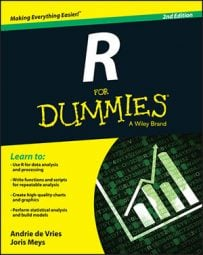##### R For DummiesGoing from a script to a function doesn’t take much effort at all. In R, a function is essentially a piece of code that is executed consecutively and without interruption. In that way, a function doesn’t differ that much from a script run using the source() function.

However, a function has two very nice advantages over scripts:

• Functions can work with variable input, so you use it with different data.

• Functions return the output as an object, so you can work with the result of that function.

The best way to learn to swim is by jumping in the deep end, so next you write a function to see how easy this is in R.

## Making the script

Suppose you want to present fractional numbers (for example, 1/2) as percentages, nicely rounded to one decimal digit. Here’s how to achieve that:

1. Multiply the fractional numbers by 100.

2. Round the result to one decimal place.

You can use the round() function to do this.

3. Paste a percentage sign after the rounded number.

4. Print the result.

The print() function does this.

You can easily translate these steps into a little script for R. So, open a new script file in your editor and type the following code:

```x <- c(0.458, 1.6653, 0.83112)
percent <- round(x * 100, digits = 1)
result <- paste(percent, "%", sep = "")
print(result)```

If you save this script as a script file — for example, pastePercent.R — you can now call this script in the console with the following command:

```> source("pastePercent.R")
 "45.8%" "166.5%" "83.1%"```

That works splendidly, as long as you want to see the same three numbers every time you call the script. But using the script for other data would be mildly inconvenient, because you would have to change the script every time.

In most editors, you also can source a script (send a complete script file to the R console) with one simple click. In RStudio, this is done by clicking the Source button or by pressing Ctrl+Shift+S for sourcing without echo, and Ctrl+Shift+Enter for sourcing with echo.

## Transforming the script

To make this script into a function, you need to do a few things. Imagine the script as a little factory that takes the raw numeric material and polishes it up to shiny percentages every mathematician will crave.

First, you have to construct the factory building, preferably with an address so people would know where to send their numbers. Then you have to install a front gate so you can get the raw numbers in. Next, you create the production line to transform those numbers. Finally, you have to install a back gate so you can send your shiny percentages into the world.

To build your factory, change the script to the following code:

```addPercent <- function(x){
percent <- round(x * 100, digits = 1)
result <- paste(percent, "%", sep = "")
return(result)
}```

Take a closer look at the different parts that make up this little factory. The function has the following elements:

• The keyword function always must be followed by parentheses. It tells R that what comes next is a function.

• The parentheses after function form the front gate, or argument list, of your function. Between the parentheses, the arguments to the function are given. In this case, there’s only one argument, named x.

• The braces, {}, can be seen as the walls of your function. Everything between the braces is part of the assembly line, or the body of your function.

• The return() statement is the back gate of your function. The object you put between the parentheses is returned from inside the function to your workspace. You can put only one object between the parentheses.

If you put all this together, you get a complete function, but R doesn’t know where to find it yet. So, you use the assignment operator <- to put this complete function into an object named addPercent. This is the address R can send numbers to for transformation. Now the function has a nice name and is ready to use.

You can’t specify in the argument list that x should be a numeric vector. For example, if you try to use a character vector as a value for x, the multiplication inside the body will throw an error because you can’t multiply characters by a number. If you want to control which type of object is given as an argument, you have to do so manually, in the body of the function.